Share

# Geometry 2013-2014 SSC (Marathi Semi-English) 10th Question Paper Solution

Subjectundefined
Year2013 - 2014 (October)
Geometry
Date: October 2013

All questions are compulsory.

1
1.i

In the given, seg BE ⊥ seg AB and seg BA ⊥ seg AD.

if BE = 6 and AD = 9 find (A(Δ ABE))/(A(Δ BAD)).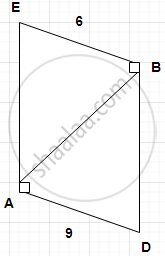Concept: Properties of Ratios of Areas of Two Triangles
Chapter:  Similarity
1.ii

If two circles having centers P and Q and radii 3 cm and 5 cm. touch each other externally, find the distance PQ.

Concept: Heights and Distances
Chapter:  Trigonometry
1.iii

The terminal is in II (second ) quadrant. what is the possible measure of an angle?

Concept: Angles in Standard Position
Chapter:  Trigonometry
1.iv

Find the slope of a line having inclination 60°.

Concept: Slope of a Line
Chapter:  Co-ordinate Geometry
1.v

Find the area of a sector of a circle having radius 6 cm and length of the arc 15 cm.

Concept: Areas of Sector and Segment of a Circle
Chapter:  Mensuration
1.vi

Sides of the triangle are 7 cm, 24 cm, and 25 cm. Determine whether the triangle is a right-angled triangle or not.

Concept: Converse of Pythagoras Theorem
Chapter:  Pythagoras Theorem
2 | Solve any four sub questions :
2.i

In the figure, ray YM is the bisector of ∠XYZ, where seg XY ≅ seg YZ, find the relation between XM and MZ.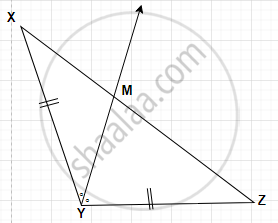Concept: Property of an Angle Bisector of a Triangle
Chapter:  Similarity
2.ii

As shown in the figure. two concentric circles are given and line AB is the tangent to the smaller circle at T. Shown that T is the midpoint of Seg AB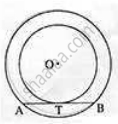Concept: Co-ordinates of the Midpoint of a Segment
Chapter:  Co-ordinate Geometry
2.iii

Find the slope of a line passing through the point A (-2,1), B (0,3).

Concept: Slope of a Line
Chapter:  Co-ordinate Geometry
2.iv

If tan θ = 2, where θ is an acute angle, find the value of cos θ.

Concept: Trigonometric Identities
Chapter:  Trigonometry
2.v

Draw the tangent at any point M on the circle with centre O and radius 2.9 cm.

Concept: Theorem - Converse of Tangent at Any Point to the Circle is Perpendicular to the Radius
Chapter:  Circle
2.vi

If (4,-3) is a point on line 5x +8y = c, find the value of c.

Concept: General Equation of a Line
Chapter:  Co-ordinate Geometry
3 | Solve any three sub-questions:
3.i

In ΔPQR, seg PM is the median. If PM = 9, PQ2 + PR2 = 290, Find QR.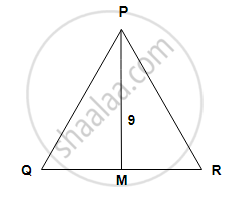Concept: Apollonius Theorem
Chapter:  Pythagoras Theorem
3.ii

In the figure, two chords AB and CD of the same circle are parallel to each other. P is the centre of the circle. show that ∠CPA = ∠DPB.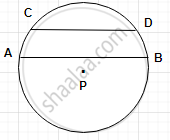Concept: Inscribed Angle
Chapter:  Circle
3.iii

Draw the circumcircle of Δ PMT in which PM = 5.4, P = 60°, M = 70°.

Concept: Problems Based on Areas and Perimeter Or Circumference of Circle, Sector and Segment of a Circle
Chapter:  Mensuration
3.iv

Prove that sqrt((1 + sin A)/(1 - sin A)) = sec A + tan A.

Concept: Trigonometric Identities
Chapter:  Trigonometry
3.v

Find the equation of a lined passage through (3,6) and making intercepts equal magnitude but opposite in sign-on both the axes.

Concept: Intercepted Arc
Chapter:  Circle
4 | Solve any two of the following sub-questions:
4.i

Prove that the lengths of two tangent segments drawn to the circle from an external point are equal.

Concept: Number of Tangents from a Point on a Circle
Chapter:  Circle
4.ii

A tree is broken by the wind. The top struck the ground at an angle of 30° and at a distance 30 m from the root. Find the whole height of the tree. (sqrt(3)=1.73)

Concept: Heights and Distances
Chapter:  Trigonometry
4.iii

The dimensions of a metallic cuboid are 44 cm × 42 cm × 21 cm. it is molten and recast into a sphere. Find the surface area of the sphere.

Concept: Introduction of Surface Areas and Volumes
Chapter:  Mensuration
5 | Solve any two sub-questions:
5.i

If the angles of a triangle are 30°, 60°, and 90°, then shown that the side opposite to 30° is half of the hypotenuse, and the side opposite to 60° is sqrt(3)/2 times of the hypotenuse.

Concept: Pythagoras Theorem
Chapter:  Pythagoras Theorem
5.ii

Δ AMT ∼ ΔAHE. In  Δ AMT, MA = 6.3 cm, ∠MAT = 120°, AT = 4.9 cm, (MA)/(HA) = 7/5. construct  Δ AHE.

Concept: Division of a Line Segment
Chapter:  Geometric Constructions
5.iii

Water flows at the rate of 10 meters per minute through a cylindrical pipe having its diameter 20 mm. how much time will it take to fill a conical vessel of diameter 40 cm and depth 24 cm?

Concept: Heights and Distances
Chapter:  Trigonometry

#### Request Question Paper

If you dont find a question paper, kindly write to us

View All Requests

#### Submit Question Paper

Help us maintain new question papers on Shaalaa.com, so we can continue to help students

only jpg, png and pdf files

## Maharashtra State Board previous year question papers 10th Geometry with solutions 2013 - 2014

Maharashtra State Board 10th undefined question paper solution is key to score more marks in final exams. Students who have used our past year paper solution have significantly improved in speed and boosted their confidence to solve any question in the examination. Our Maharashtra State Board 10th undefined question paper 2014 serve as a catalyst to prepare for your Geometry board examination.
Previous year Question paper for Maharashtra State Board 10th undefined-2014 is solved by experts. Solved question papers gives you the chance to check yourself after your mock test.
By referring the question paper Solutions for Geometry, you can scale your preparation level and work on your weak areas. It will also help the candidates in developing the time-management skills. Practice makes perfect, and there is no better way to practice than to attempt previous year question paper solutions of Maharashtra State Board 10th.

How Maharashtra State Board 10th Question Paper solutions Help Students ?
• Question paper solutions for Geometry will helps students to prepare for exam.
• Question paper with answer will boost students confidence in exam time and also give you an idea About the important questions and topics to be prepared for the board exam.
• For finding solution of question papers no need to refer so multiple sources like textbook or guides.
S